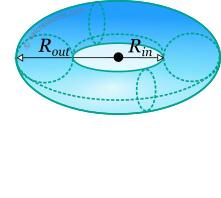Jump to
Table of Contents
Share this

## Properties of Torus

This tool calculates the basic geometric properties of a ring torus. Enter the shape dimensions Routand Rinbelow. The calculated results will have the same units as your input. Please use consistent units for any input.

 Rout = Rin =
 Volume = Surface area =Table of Contents
Share this

## Definitions

### Geometry

The torus is a 3-dimensional object, that can be created through rotation a circular disc around a circle. The volume of a torus is given by the formula:

where the outer radius of the torus and its inner radius.

The surface area of the torus is given by the next formula: